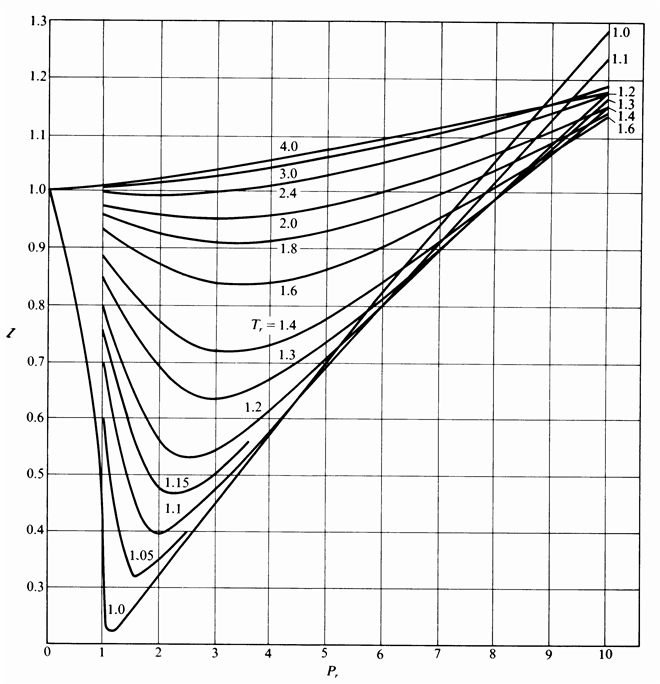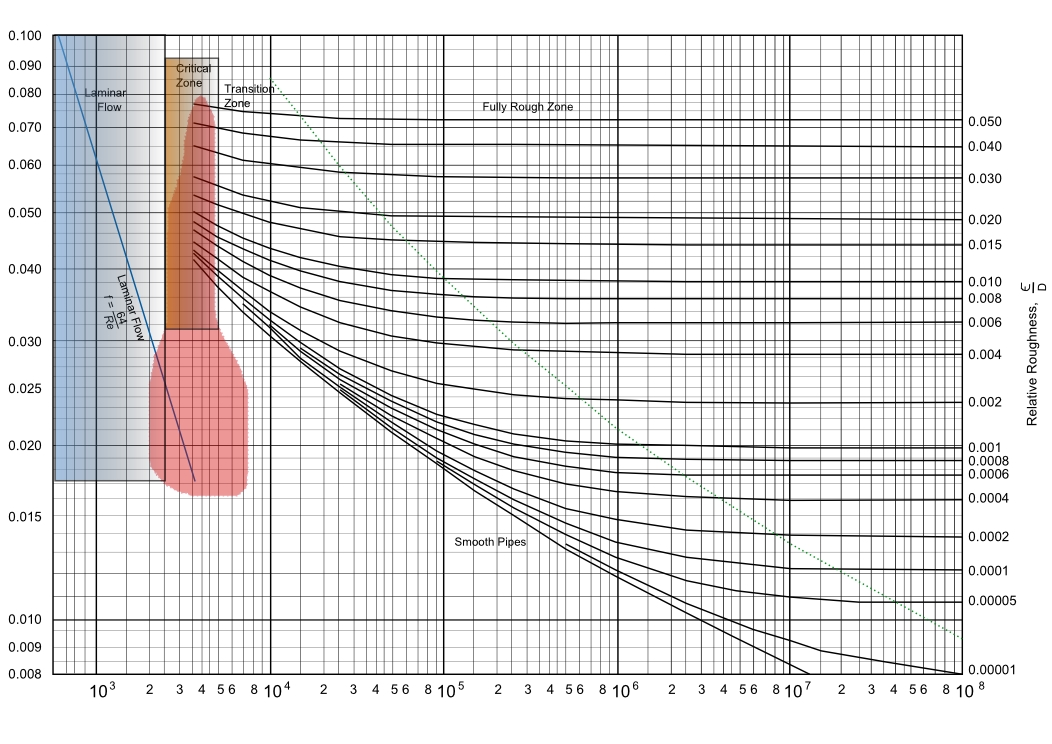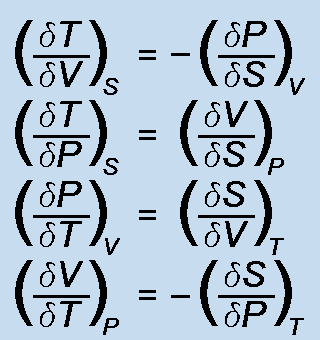### ChemE Soft StoreCaCO3 -> CaO + CO2
NaCl + NH3 + CO2 + H2O -> NaHCO3 + NH4Cl
2 NaHCO3 -> Na2 CO3 + H2O + CO2
CaO + H2 O -> Ca(OH)2
Ca(OH)2 + 2 NH4 Cl -> CaCl2 + 2 NH3 + 2 H2O

Pressure Loss

The fluid flow friction factor is calculated from the Reynolds number and pipe roughness using Moody's definitions. Pressure drop is then calculated for the flowing fluid in the pipe, which may include elbows.

Meter Calcs

Venturi and orifice meters are designed for size, flow rate and pressure drop. Calculations for velocity through the meter, Reynolds number, discharge coefficient and expansion factor are shown. Fluids include both non-compressible and compressible fluids.

### Heat Transfer

Heat transfer rates, both conductive and convective, are calculated using thermal properties of material (conductive), or fluid (convective).